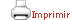Teaching plan for the course unit

(Short version)

 Català English CloseGeneral information

Course unit name: Generalized Linear Models

Course unit code: 361234

Coordinator: JORDI CORTES MARTINEZ

Department: Faculty of Economics and Business

Credits: 6

Single program: S

 Estimated learning time Total number of hours 150

 Face-to-face and/or online activities 60
 -  Lecture with practical component Face-to-face and online 30 -  IT-based class Face-to-face and online 30
 Supervised project 40
 Independent learning 50

 Learning objectives
 Referring to knowledge — Understand the processes of parameter estimation in a generalized linear model. — Know how to characterize a linear regression model with continuous, binary and integer response (using either direct counting or log-linear models) — Know the statistical indicators of the goodness of fit and its validity for the diagnosis and validation of the linear models proposed, at the level of prediction. — Know how to validate a generalized linear model. — Know how to interpret the inferential results derived from the fit of a generalized linear model.  Referring to abilities, skills — Know how to estimate the parameters of a generalized linear model. — Know how to analyse the main hypothesis tests associated with generalized linear models. — Know how to carry out goodness-of-fit tests in adjusted generalized linear models. — Know how to verify whether the adjusted model fulfils the assumptions of the family of distributions used. — Know how to solve some frequently applied generalized linear models: general linear model, logistic regression and log-linear models. — Be able to interpret the results rigorously. — Be able to choose between the various possibilities provided by a statistical package, both in terms of modeling capabilities and information output, in order to be able to draw useful conclusions in the specific modeling process being undertaken.

 Teaching blocks

1. Introduction to generalized linear models

1.1. Introduction: models in general and the need to extend linear models

1.2. Definition of the generalized linear model (GLM): Random component: family and parameters. Deterministic component: linear predictor and link function

1.3. GLMs with normal distribution: properties and examples

1.4. Common families of distribution in GLMs: Gaussian, gamma, inverse Gaussian, Bernoulli, binomial, Poisson, negative binomial

1.5. Parameter estimation

1.6. Quasi-likelihood

2. GLMs: inference

2.1. Predictions. Measures of goodness of fit: deviance, generalized Pearson statistic

2.2. Estimation of the dispersion parameter ¿ when it is unknown

2.3. Residual analysis

2.4. Estimate properties and goodness-of-fit tests of fitted models

2.5. Examples of GLMs with a continuous response variable

3. Binary response models

3.1. Logistic regression: binomial response

3.2. Interpretation of usual links (logit, probit and cloglog)

3.3. Estimation, inference and validation

3.4. Predictive capacity measures

3.5. Presentation of case studies

4. Count models

4.1. Poisson, quasi-Poisson and negative binomial regression models

4.2. Diagnosis and treatment of over-dispersion

4.3. Zero-inflated and zero-truncated models

4.4. Log-linear models: Modeling of contingency tables

4.5. Estimation, inference and validation

4.6. Presentation of case studies

Book

McCULLAGH, Peter, et al. Generalized linear models. London [etc.]: Chapman & Hall, 1989

FOX, John. Applied Regression Analysis and Generalized Linear Models. Los Angeles [etc.]: SAGE, 2008

FOX, John, et al. An R Companion to Applied Regression. Thousand Oaks, Calif.: SAGE, 2011

DOBSON, Annette J. An Introduction to generalized linear models. Boca Raton: CRC Press / Chapman & Hall, 2008

FARAWAY, Julian James. Extending the Linear Model with R, Generalized Linear, Mixed Effects and Nonparametric Regression Models. Boca Raton (Mass.): Chapman & Hall/CRC, 2006

ZUUR, Alain F et al. Mixed Effects Models and Extensions in Ecology with R. New York (NY): Springer, 2009

Web page

The R Project for Statistical Computing